## Screenshots

•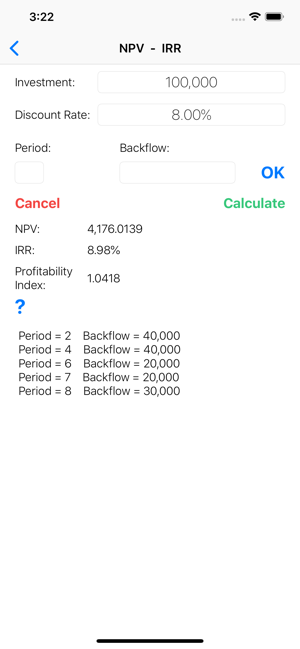•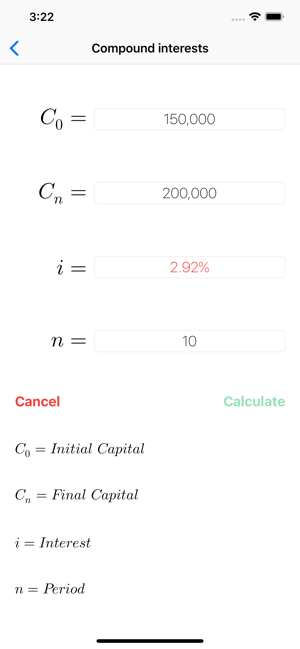•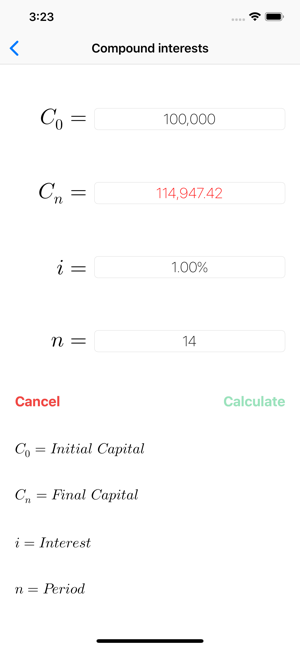•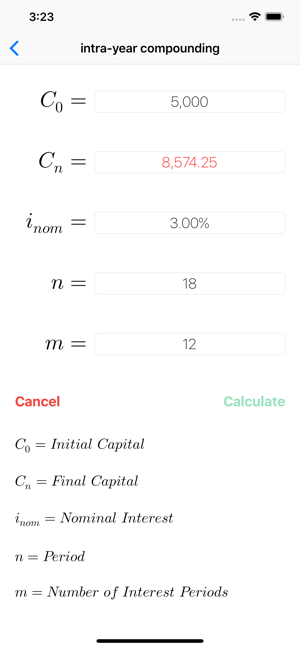•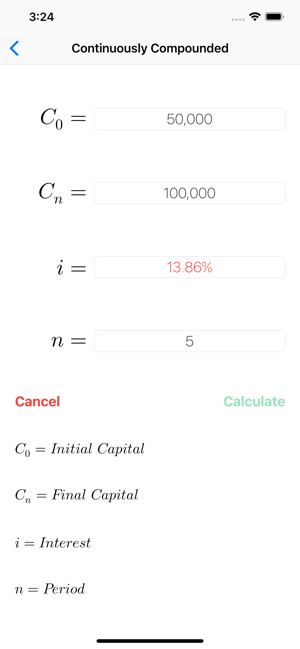•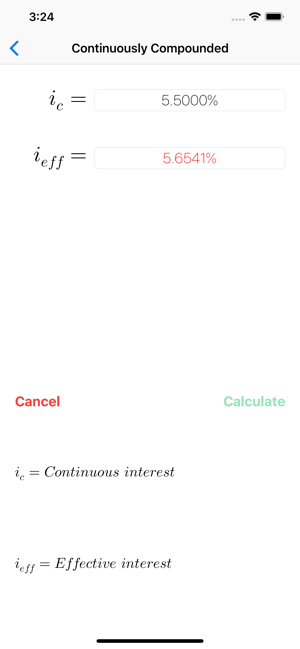•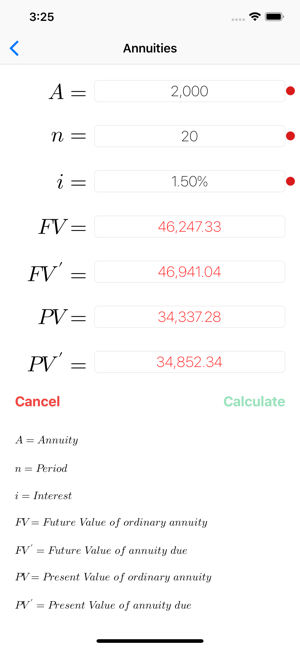•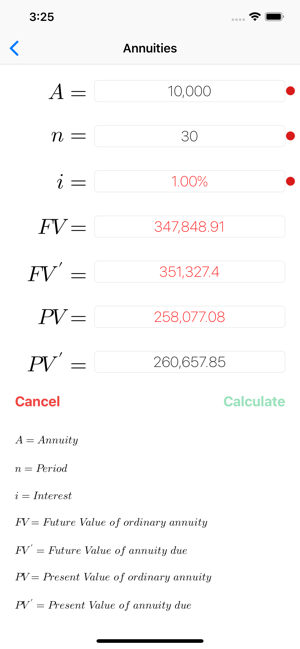•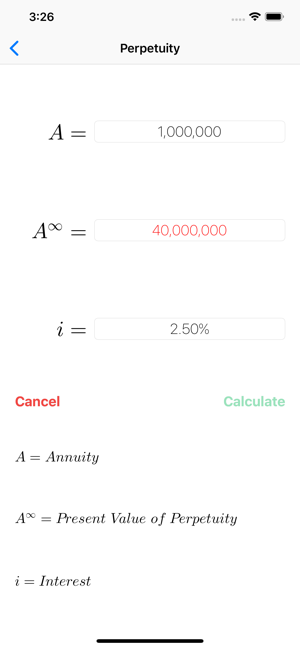•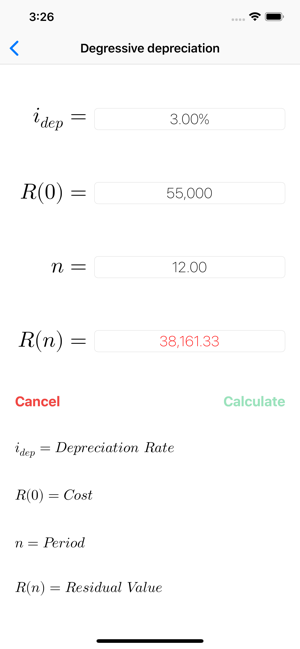## Description

Financial mathematics calculator, very easy to use.
The App can solve equations. In the screenshots the user input is black, the calculated data are red.
FinCalcPro CAN SOLVE EVERY VARIABLE OF THE ABOVE LIST !!!

The following types of calculation are supported:

• PERCENTAGES
- calculation of the percentage (augmentation / reduction)
- calculation of the previous value
- calculate of the new value

• LINEAR INTEREST
- calculation of the initial capital
- calculation of the end capital
- calculation of the interest
- calculation of the period

• COMPOUND INTEREST
- calculation of the initial capital
- calculation of the end capital
- calculation of the interest
- calculation of the period

• INTRA-YEAR COMPOUNDING
- calculation of the initial capital
- calculation of the end capital
- calculation of the interest
- calculation of the period
- calculation of the number of intra-year periods
-conversion nominal interest / effective interest
- conversion effective interest / nominal interest

• CONTINUOUSLY COMPOUNDED INTEREST
- calculation of the initial capital
- calculation of the end capital
- calculation of the interest
- calculation of the period
-conversion continuous interest rate / effective interest rate
- conversion effective interest rate / continuous interest rate

• ORDINARY ANNUITIES AND ANNUITIES DUE
- calculation of the annuity
- calculation of the interest
- calculation of the final value
- calculation of the present value

• PERPETUITIES
- calculation of the annuity
- calculation of the interest
- calculation of the present value

• LINEAR DEPRECIATION
- calculation of the depreciation rate
- calculation of the new value
- calculation of the residual value

• DEGRESSIVE DEPRECIATION
- calculation of the depreciation rate
- calculation of the new value
- calculation of the residual value

• NPV - IRR
- calculation of the Net Present Value (NPV)
- calculation of the Internal Rate of Return (IRR)
- calculation of the Profitability Index

## What’s New

Version 2.4

Language settings
Minor bug fixes
New icon

## Ratings and Reviews

1.8 out of 5
4 Ratings

4 Ratings

Makita_K ,

### Can’t find language setting

Can’t change language.

### Developer Response ,

With the new update is possible to change language.

Andrey AV ,

### Can't change language on English

Can't change language on English

### Developer Response ,

With the new update is possible to change language in English.

## Information

Seller
Francesco Grassi
Size
3.7 MB
Category
Finance
Compatibility

Requires iOS 9.0 or later. Compatible with iPhone, iPad, and iPod touch.

Languages

English, French, German, Italian

Age Rating
4+
Price
\$2.99

## Supports

•